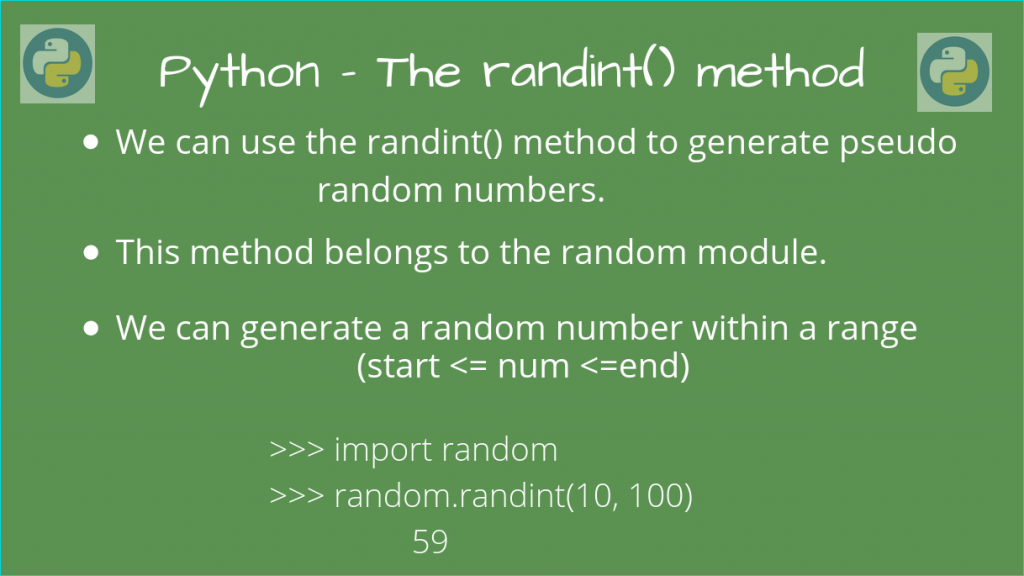# Generate random integers using Python randint()In this article, we’ll take a look at generating random integers using the Python randint() method.

This method is in the random module in Python, which we will use to generate pseudo-random numbers, so we’ll need to import it to load this method. Let’s take a look at this method now!

## Syntax of Python randint()

The Python randint() method returns a random integer between two limits `lower` and `upper`(inclusive of both limits). So this random number could also be one of the two limits.

We can call this function as follows:

```random_integer = random.randint(lower, upper)
```

Here, lower is the lower limit of the random number, and upper is the upper limit of the random number.

We must ensure that `lower` and `upper` are integers, and that `lower` <= `upper`. Otherwise, a `ValueError` Exception will be raised.

Let’s take a look at how we can use this function now.

## Using the Python randint() method

We’ll need to import the `random` module. After that, we can call the function using the syntax.

```import random

beg = 10
end = 100

# Generates a random integer between (10, 100)
random_integer = random.randint(beg, end)

print(f"A random integer between {beg} and {end} is: {random_integer}")
```

Possible Output

```A random integer between 10 and 100 is: 59
```

Indeed, we can see that this number does lie between the range (10, 100).

If we want to repeat this pseudo-random generation, let’s use a loop for that.

```import random

beg = 0
end = 100

rand_list = [random.randint(beg, end) for _ in range(10)]

print(rand_list)
```

Possible Output

```[61, 16, 39, 86, 25, 11, 16, 89, 99, 70]
```

We can see that these numbers are in the 0 to 100 range. And the pseudo-random conditions indicate that no two consecutive numbers repeat.

NOTE: As I mentioned earlier, both `beg` and `end` must be integers, with `beg` <= `end`. Otherwise, we will get a `ValueError` Exception.

## Conclusion

In this article, we learned how we could use the `randint()` method in Python, to generate random integers.